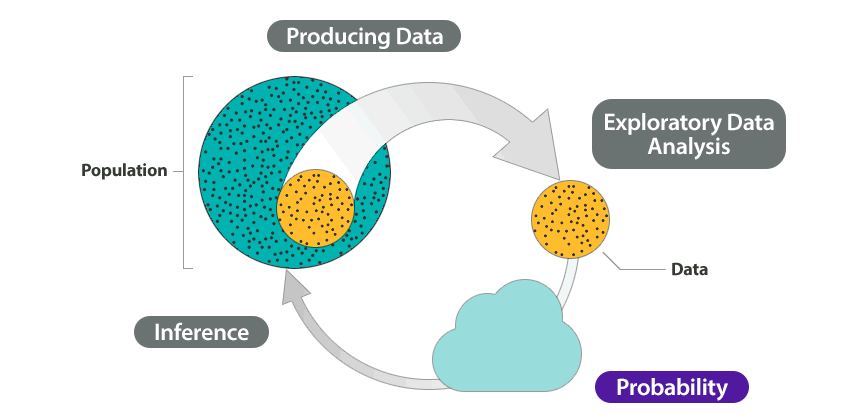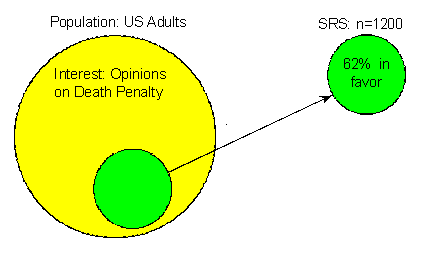## Why It Matters: Probability and Probability Distributions

Recall the Big Picture—the four-step process that encompasses statistics (as it is presented in this course):So far, we’ve discussed the first two steps:

Producing data—how data are obtained and what considerations affect the data production process.

Exploratory data analysis—tools that help us get a first feel for the data by exposing their features using graphs and numbers.

Our eventual goal is inference—drawing reliable conclusions about the population on the basis of what we’ve discovered in our sample. To really understand how inference works, though, we first need to talk about probability, because it is the underlying foundation for the methods of statistical inference. We use an example to try to explain why probability is so essential to inference.

First, here is the general idea: As we all know, the way statistics works is that we use a sample to learn about the population from which it was drawn. Ideally, the sample should be random so that it represents the population well.

Recall from Types of Statistical Studies and Producing Data that when we say a random sample represents the population well, we mean that there is no inherent bias in this sampling technique. It is important to acknowledge, though, that this does not mean all random samples are necessarily “perfect.” Random samples are still random, and therefore no random sample will be exactly the same as another. One random sample may give a fairly accurate representation of the population, whereas another random sample might be “off” purely because of chance. Unfortunately, when looking at a particular sample (which is what happens in practice), we never know how much it differs from the population. This uncertainty is where probability comes into the picture. We use probability to quantify how much we expect random samples to vary. This gives us a way to draw conclusions about the population in the face of the uncertainty that is generated by the use of a random sample. The following example illustrates this important point.

## Death Penalty

Suppose we are interested in estimating the percentage of U.S. adults who favor the death penalty. To do so, we choose a random sample of 1,200 U.S. adults and ask their opinion: either in favor of or against the death penalty. We find that 744 of the 1,200, or 62%, are in favor. (Although this is only an example, 62% is quite realistic given some recent polls). Here is a picture that illustrates what we have done and found in our example:Our goal is to do inference—to learn and draw conclusions about the opinions of the entire population of U.S. adults regarding the death penalty on the basis of the opinions of only 1,200 of them.

Can we conclude that 62% of the population favors the death penalty? Another random sample could give a very different result, so we are uncertain. But because our sample is random, we know that our uncertainty is due to chance, not to problems with how the sample was collected. So we can use probability to describe the likelihood that our sample is within a desired level of accuracy. For example, probability can answer the question, How likely is it that our sample estimate is no more than 3% from the true percentage of all U.S. adults who are in favor of the death penalty?

Answering this question (which we do using probability) is obviously going to have an important impact on the confidence we can attach to the inference step. In particular, if we find it quite unlikely that the sample percentage will be very different from the population percentage, then we have a lot of confidence that we can draw conclusions about the population on the basis of the sample.

In this module, we discuss probability more generally. Then we begin to develop the probability machinery that underlies inference.